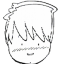This topic is locked
[SOLVED]

add days month and years date field

10/13/2021 3:53:12 PM
L

Hello dear, I am trying to do a preventive maintenance system, and I want to update a task (edit) to modify the date of the next task.
I tried with a code that I found in the forum which is the following

\$ values ["Date"] = date ("Y-m-d", strtotime (\$ values ["Date"]. '+ 30 days'));

but then when modifying it as I need it so that the number of days is variable from an element in the same table it does not work,

\$ values ["Date"] = date ("Y-m-d", strtotime (\$ values ["Date"]. '+'. \$ values ["Repeat"]. 'days'));

Repetition has 1, 15, 30, 180, 360 days.

Also add a value called a period that has days, months, and years. but I still do not add it since I am stuck with the days.

if you come up with an idea it will be welcome. From already thank you very much!

7 replies
A
acpan 10/13/2021
 Try enquote your string like this:\$values["Date"] = date("Y-m-d", strtotime (' " ' +\$values["Date"]. '+ '. \$values["Repeat"]. ' days' + ' " ') );Alternately, you can form the \$next_date as string first then apply the strtotime funciton for ease of troubleshooting: :// Verify your date value, in case it is invalid:echo \$values["Date"]; // should be proper date format \$next_date = \$values["Date"]. '+ '. \$values["Repeat"]. ' days';echo \$next_date; // Sample data: 2020-10-10 +5 days\$values["Date"] = date("Y-m-d", strtotime (\$next_date) ) ;
L
 hello thank you very much for answering!I tested your code and after several tests and changes, I managed to get the following code to work,\$myDate= \$values["Fecha"];\$nDays= 15;\$DateMy= strtotime ( \$myDate. '+' .\$nDays. 'days');\$values["Fecha"]= date('Y-m-d', \$DateMy);but when in \$ nDays I add the following, \$ nDays = \$ values ['Repeat']; It doesn't work, I don't know what I'm doing wrong.in the mysql database I tried that the value Repeat is int and varchar. and it does not work.\$myDate= \$values["Fecha"];\$nDays= \$values['Repeticion'];\$DateMy= strtotime ( \$myDate. '+' .\$nDays. 'days');\$values["Fecha"]= date('Y-m-d', \$DateMy);thanks greetingsMyr0n 10/15/2021
 maybe the value in \$values['Repeticion'] is null or something different than a number if that is the case convert the value in integer like the next code\$myDate= \$values["Fecha"];\$nDays= (int) \$values['Repeticion'];\$DateMy= strtotime ( \$myDate. '+' .\$nDays. 'days');\$values["Fecha"]= date('Y-m-d', \$DateMy);
A
acpan 10/15/2021
 +1 to myrOn's suggestion. You are very close and i think the issue is with \$values['Repeticion"] by taking the isolation approach suggested. Echo it out and see the monster.
L